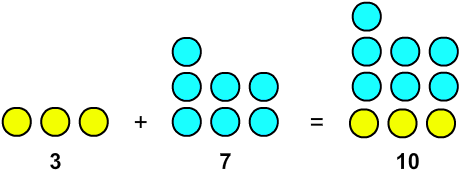Rate 0 stars Common Core
Quiz size:
Message preview:
Someone you know has shared Addition Sentences - True/False quiz with you:

To play this quiz, click on the link below:

Hope you have a good experience with this site and recommend to your friends too.

Login to rate activities and track progress.
Login to rate activities and track progress.

If the two sides of an addition sentence are equal, then the sentence is said to be true.

For example: Consider the addition sentence.

3 + 7 = 7

Let's check if the given addition sentence is true.

Find the sum of 3 and 7.3 + 7 is not equal to 7.

So, the given addition sentence 3 + 7 = 7 is not true.

ds

A B C D E F G H I J K L M N O P Q R S T U V W X Y Z

### Help

##### Remember :

The smallest number is the one that comes first while counting.

##### Solution :

To arrange the given numbers in order from smallest to greatest, find the smallest number among all the given numbers.

21,27,23

21 is the smallest number.